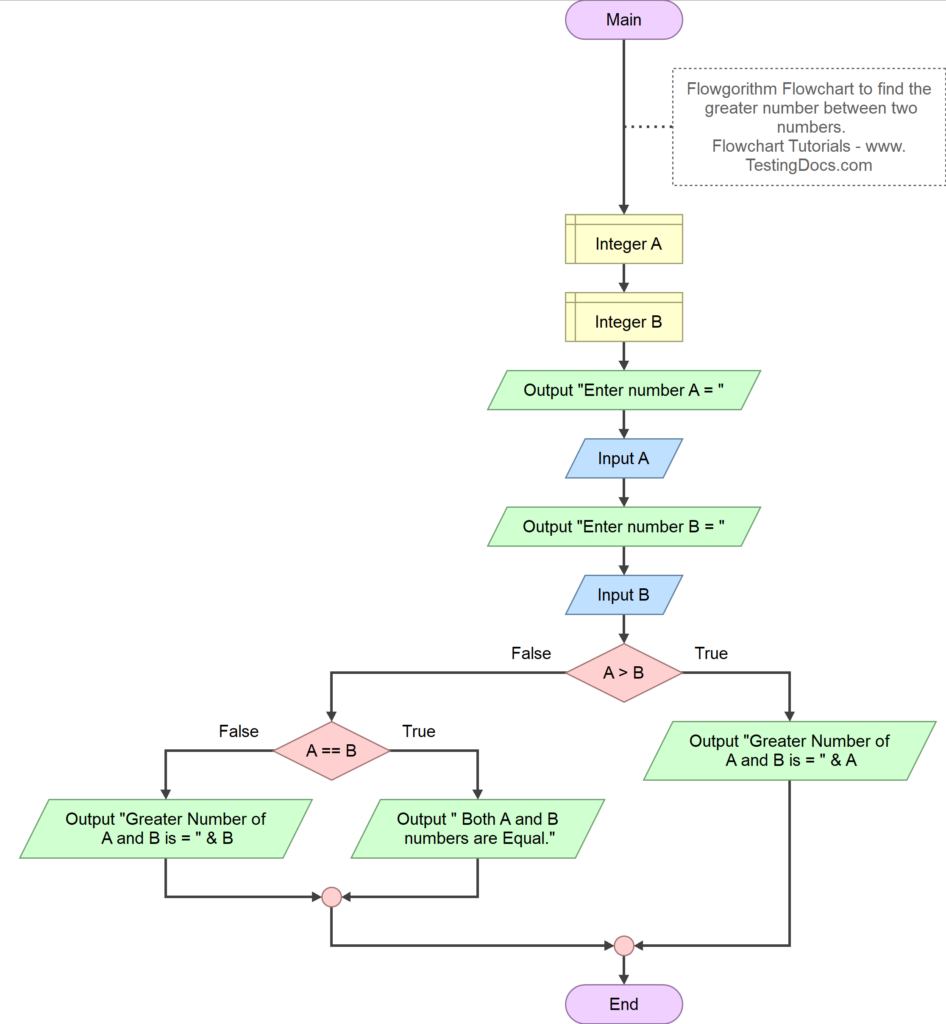# Flowchart Tutorials

## What is a Flowchart?

First things first, A flowchart is a graphical representation of an algorithm. It represents a graphic modelling language to represent the flow of control and execution of algorithms, procedures, etc.

Flowchart allows you to describe in a graphic way the sequence of steps and instructions in which they must be performed. Everything in a flowchart is represented by a geometric figure.

## What is an Algorithm?

When designing an algorithm we need to consider the architecture of the computer. Computer architectures can be sequential or parallel.

## Sequential Algorithm

An algorithm is a sequence of steps that are executed to solve the problem. The algorithm steps should be precise and the result should be obtained after execution of finite number of the steps. i.e an algorithm should halt after finite amount of time. There can be multiple ways to solve a problem. An efficient algorithm should take less amount of space and time.

An algorithm can be analyzed mostly on two factors:

• Time Complexity – Execution Time taken by the algorithm.
• Space Complexity – Amount of Space required by the algorithm.

## Parallel Algorithm

Time and mission critical systems need faster output. These systems make use of parallel algorithms. A parallel algorithm can execute several steps concurrently on different processors and then combine all the individual results to produce the final output.

## Algorithm Example

Algorithm to find the greater number between two numbers

```START
Declare Integer A
Declare Integer B

OUTPUT "Enter number A = "
INPUT A
OUTPUT "Enter number B = "
INPUT B
IF A > B
OUTPUT "Greater Number of A and B is = " & A
ELSE
IF A == B
OUTPUT " Both A and B numbers are Equal."
ELSE
OUTPUT "Greater Number of A and B is = " & B
END
END
END```

## Flowchart Symbols

The flowchart symbols represent the flowchart statements or steps. Everything in a flowchart is represented by a geometric figure. They are shown with various kinds of figures like parallelogram, diamonds, circle, etc. They are connected with arrow headed flow lines to show the program control.

https://www.testingdocs.com/flowchart-symbols/

## Flowchart Example

Flowchart to find the greater number between the two given numbers A and B.

Flowchart example developed using Flowgorithm flowchart software application.## Free Flowchart Software

Most Flowcharts on this website use the below free flowchart software tools.

• RAPTOR
• Flowgorithm

## RAPTOR

https://www.testingdocs.com/raptor-a-flowchart-tool/

## Flowgorithm

Flowgorithm is a free application that allows you to create flowchart programs

https://www.testingdocs.com/flowgorithm-flowchart-tutorial/